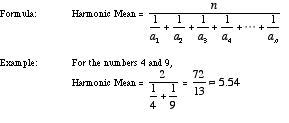index: click on a letter A B C D E F G H I J K L M N O P Q R S T U V W X Y Z A to Z index index: subject areas numbers & symbols sets, logic, proofs geometry algebra trigonometry advanced algebra & pre-calculus calculus advanced topics probability & statistics real world applications multimedia entrieswww.mathwords.com about mathwords website feedback

 Harmonic Mean A kind of average. To find the harmonic mean of a set of n numbers, add the reciprocals of the numbers in the set, divide the sum by n, then take the reciprocal of the result. The harmonic mean of {a1, a2, a3, a4, . . ., an} is given below.See also Mean